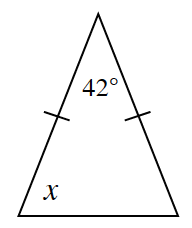### Home > GC > Chapter 5 > Lesson 5.3.4 > Problem5-105

5-105.

Multiple Choice: In the triangle below, $x$ must be:

1. $42º$

1. $69º$

1. $21º$

1. $138º$

1. none of theseThe triangle is isosceles meaning $2$ sides are equal.

The measure of $x$ must be the same as the other unknown angle because base angles are equal in an isosceles triangle.

Since the sum of all the interior angles of a triangle must be $180º$, then $x+x+42º=180º$.